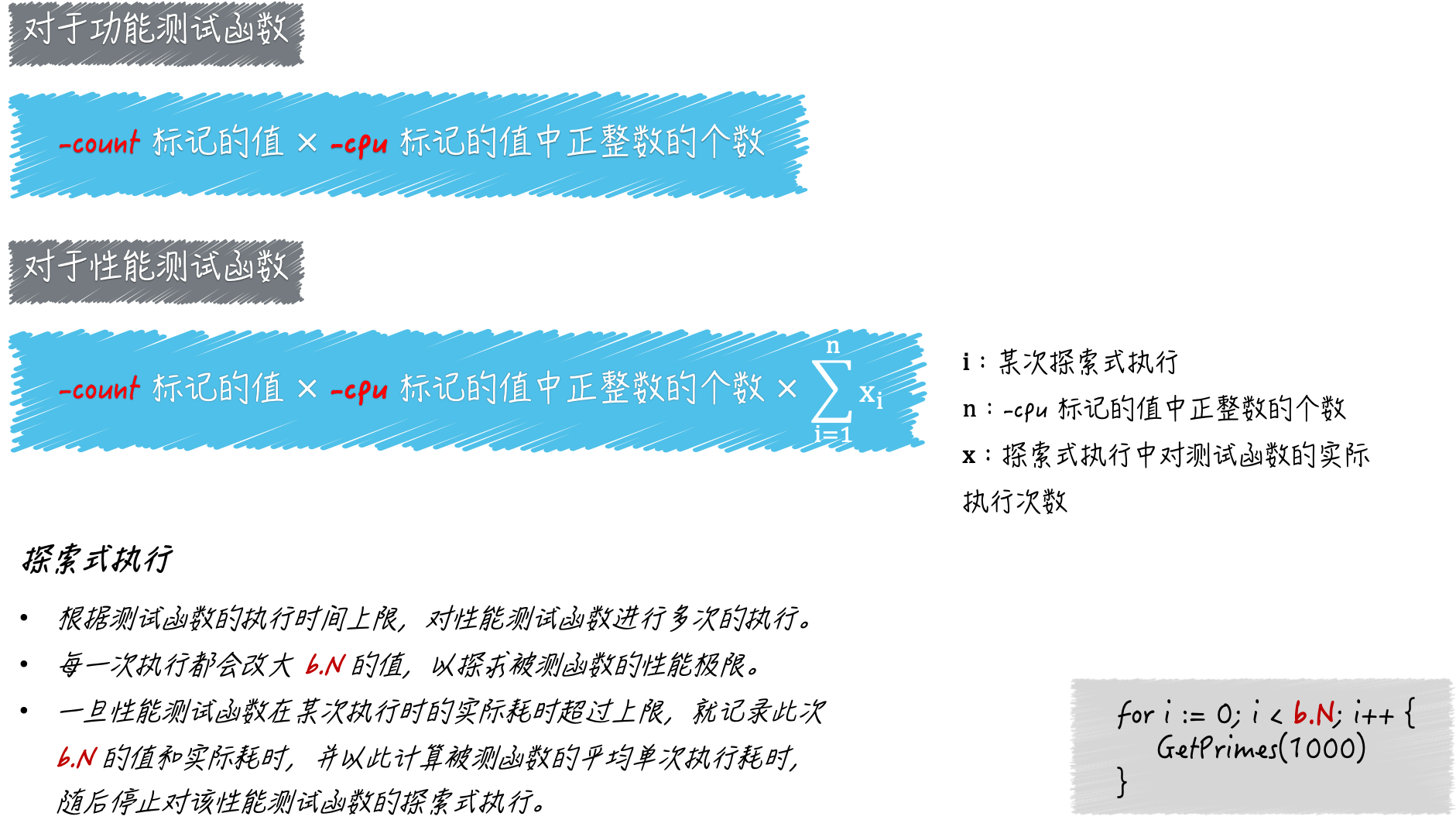# Go语言核心36讲-更多的测试手法

### 前导内容：-cpu 的功能

go test命令的标记-cpu，它是用来设置测试执行最大 P 数量的列表的。

Go 语言并发编程模型中的 P，正是 goroutine 的数量能够数十万计的关键所在。P 的数量意味着 Go 程序背后的运行时系统中，会有多少个用于承载可运行的 G 的队列存在。

### 今天的问题是：怎样设置-cpu标记的值，以及它会对测试流程产生什么样的影响？

#### 问题解析

go test命令在进行准备工作的时候会读取-cpu标记的值，并把它转换为一个以int为元素类型的切片，我们也可以称它为逻辑 CPU 切片。

``性能测试函数的执行次数 = `-cpu`标记的值中正整数的个数 x `-count`标记的值 x 探索式执行中测试函数的实际执行次数``

``功能测试函数的执行次数 = `-cpu`标记的值中正整数的个数 x `-count`标记的值``### 知识扩展

#### 问题 2：性能测试函数中的计时器是做什么用的？

``````// demo.go
package q3

import (
"math"
)

// GetPrimes 用于获取小于或等于参数max的所有质数。
// 本函数使用的是爱拉托逊斯筛选法（Sieve Of Eratosthenes）。
func GetPrimes(max int) []int {
if max <= 1 {
return []int{}
}
marks := make([]bool, max)
var count int
squareRoot := int(math.Sqrt(float64(max)))
for i := 2; i <= squareRoot; i++ {
if marks[i] == false {
for j := i * i; j < max; j += i {
if marks[j] == false {
marks[j] = true
count++
}
}
}
}
primes := make([]int, 0, max-count)
for i := 2; i < max; i++ {
if marks[i] == false {
primes = append(primes, i)
}
}
return primes
}

//demo_test.go
package q3

import (
"testing"
"time"
)

func BenchmarkGetPrimes(b *testing.B) {
// 你可以注释或者还原下面这四行代码中的第一行和第四行，
// 并观察测试结果的不同。
b.StopTimer()
time.Sleep(time.Millisecond * 500) // 模拟某个耗时但与被测程序关系不大的操作。
max := 10000
b.StartTimer()

for i := 0; i < b.N; i++ {
GetPrimes(max)
}
}``````

上一篇Go语言核心36讲-sync.Mutex与sync.RWMutex

2021-11-23Go语言核心36讲-测试的基本规则和流程（上）

2021-11-23
目录